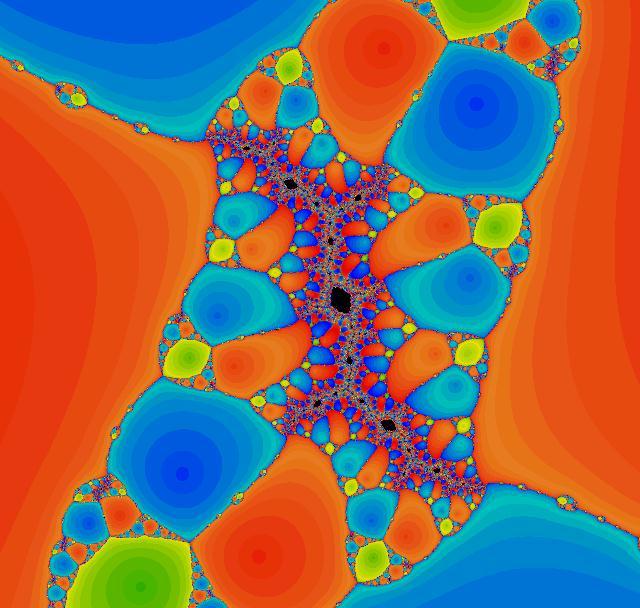# Newton on Cubics

The cubic polynomial is (x-1)(x-(-1/2 + a))(x-(-1/2 - a))

roots are centered at the origin

first three critical points of Newton's method are the roots

last critical point is at 0.

The large-scale parameter picture obtained by plotting rate of attraction of 0 to 3 roots.

• Red=1
• Green=-1/2+a
• Blue=-1/2-a
• Black=none of the above.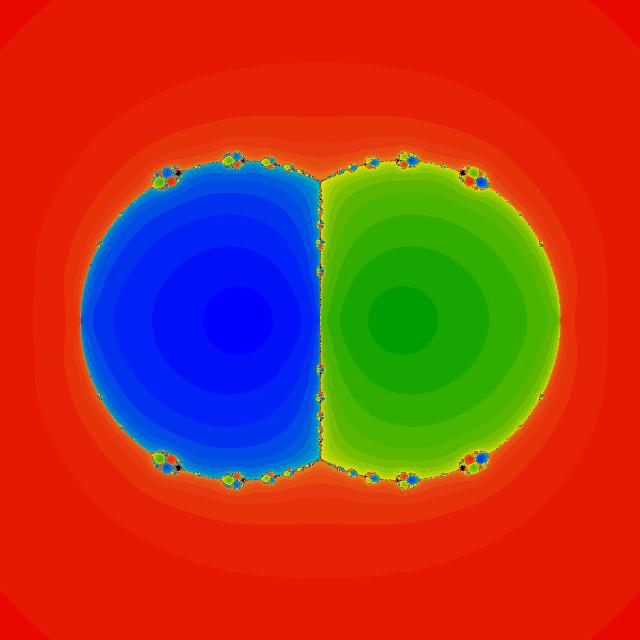First Blowup "The Mandelbrain"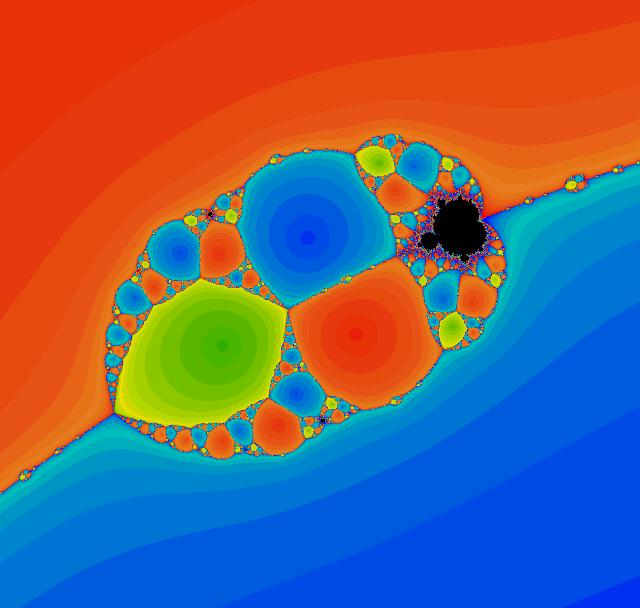Second Blowup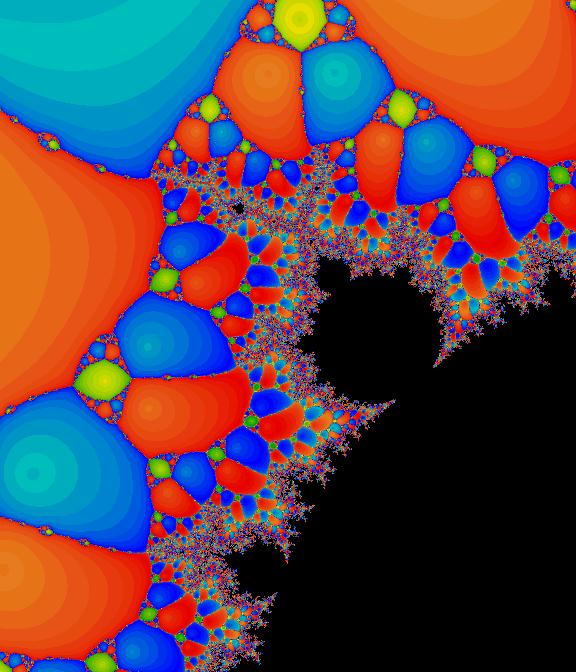J-set corresponding to the baby mandelbroty in the above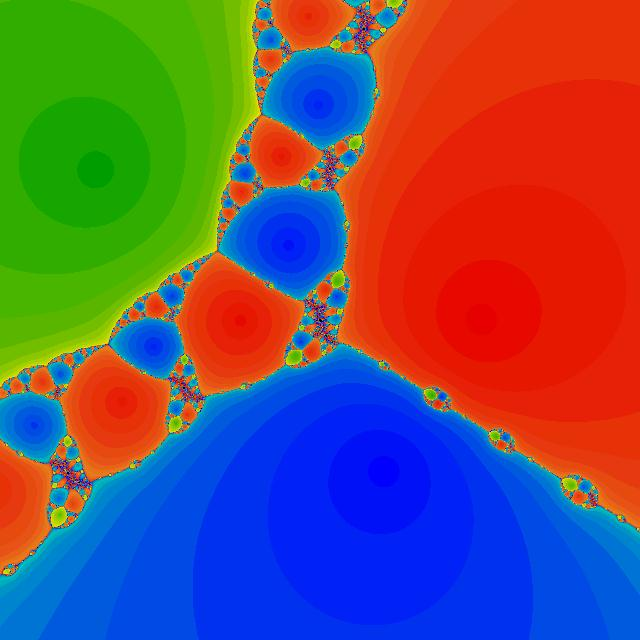Blowup of J-set